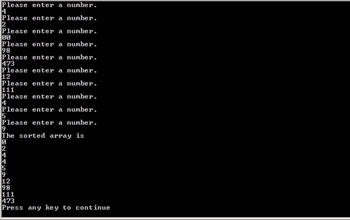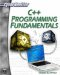# Algorithms

Computer programs are used to solve problems; that is the entire reason people write computer programs. Unfortunately, many beginning computer programmers approach computer programming in an ad hoc manner, simply cobbling together a patchwork of code that hopefully will properly process their data. There is a much more efficient way to solve problems. This method is called an algorithm. An algorithm is a formal methodology for solving a problem. It is a step-by-step recipe for performing some action. When you take a mathematics course in high school or college, you are taught a number of algorithms—methods for solving problems. In math, these problems involve finding the slope of an equation, finding the length of one side of a triangle, or using integral calculus to calculate the area of function. Another way to phrase that definition of an algorithm is to say that an algorithm is a precise definition of a computation.

Computer scientists study algorithms and the efficacy of algorithms. In a standard baccalaureate computer science degree, students spend an entire semester studying and analyzing algorithms. In graduate-level computer science programs, a deeper study of algorithms is often pursued. That level of algorithm study is well beyond the scope of this book, however we will spend some time studying some of the most common algorithms.

One of the most common algorithms is the sorting algorithm. The need to sort a list is a very common problem. For example, sorting a list of phone numbers into either ascending or descending order is often necessary. Due to the commonality of this problem, computer scientists spend a great deal of time studying sorting algorithms. For our purposes, we will examine the two most common algorithms. These are the bubble sort and the quick sort.

### Bubble Sort

The bubble sort is a simple but effective method for sorting a list of numbers. The bubble sort starts at the beginning of the list. Each element is compared to the element next to it. If the element on the left is larger than the element on the right, then the two numbers are switched. The process is continued until all numbers are in order. It might be helpful to examine an actual code example. In this example, you will see a larger than usual number of comments. It is hoped that the additional commenting will help you to better understand the example.

### Example 13.3

Step 1: Enter the following code into a text editor.

`#include <iostream> using namespace std; int main() {  int numbers,temp,i,size = 10;  int original,sorted;  //Creates an array of 10 user supplied numbers.  for(i=0;i<size;i++) {   cout<<"Please enter a number.\n";   cin>>numbers[i]; } // end of for loop //outer loop goes through the entire list for(original=1; original < size; original++) {   for(sorted = size-1; sorted >= original; sorted—)   { /*If element sorted-1 is greater than element after  it then swap it.*/ if(numbers[sorted-1] > numbers[sorted])      {         temp = numbers[sorted-1];    numbers[sorted-1] = numbers[sorted];    numbers[sorted] = temp;      }// end of if        }// end of inner for loop }// end of outer for loop //print the sorted array. cout << "The sorted array is \n"; for(i=0;i<size;i++) {  cout<<numbers[i]<<" "<<endl; }//end of the for loop    return 0; }// end of main`

Step 2: Compile the code and execute it. You should see something much like what is displayed in Figure 13.4.Figure 13.4: The bubble sort.

Notice that the first portion of the program is getting the user to enter in 10 integers. The sorting portion is done by a nested loop structure. This is something you may not have previously encountered. A nested loop is a loop that is inside another loop. Each time the outer loop executes, the inner loop will go through its complete cycle. This means that this algorithm has to execute a number of times equal to the maximum of the outer loop, times the maximum of the inner loop. This is important because when one studies algorithms, a great deal of attention is devoted to which algorithm is most effective, which one can execute the quickest.

The second loop in the bubble sort holds the key to this algorithm.

` if(numbers[sorted-1] > numbers[sorted])`

The statement simply says that if the number in element i-1 is greater than the number located in element i, then swap them. So if the third element of the array is 5 and the fourth element of the array is 2, then swap them to put them in order. If you wanted to sort the array into descending array instead, then change the greater than in the if statement to a less than.

### Quick Sort

Bubble sort and quick sort are the two most commonly encountered sorting algorithms. That’s not to say that they are the best sorting algorithms, but that they are the most commonly encountered. The quick sort is based on the idea of splitting an array down the middle and sorting both sides, then putting them back together. If both sections are sorted simultaneously then this should, theoretically, sort faster. The following example is commented rather heavily in an attempt to facilitate your understanding of the code presented. There will also be some commentary after the example.

### Example 13.4

Step 1: Enter the following code into your favorite text editor.

`#include <iostream> using namespace std;   // function prototypes int *swapnumbers(int rawdata[], int lower, int upper); int *quicksort(int rawdata[], int first, int last); int main() {      int unsorted,i;      int *sorted;// pointer to a sorted array      // enter numbers in array in       // any order at all.      for(i = 0;i<10;i++)      {    cout << "Please enter an integer \n";      cin >> unsorted[i];      }      // call the quick sort function.      // Pass it the unsorted array and the starting      // number of the array.  Also pass it the size of      // the array.      sorted = quicksort(unsorted, 0, 10);      // print out the sorted array      cout << "This is your array sorted:"<< endl; for(i = 0; i < 10; i++) {      cout << sorted[i] << endl; }      return 0; } int *quicksort(int rawdata[], int first, int last) {      int lower = first+1, upper = last;      int bound;      // begin by swapping numbers       // the first+last /2 gives you the middle      // point of the array...often called the      // pivot point      rawdata = swapnumbers(rawdata, first, (first+last)/2);      bound = rawdata[first];            // break the array into two partitions      while(lower <= upper)       {      while(rawdata[lower] < bound)      lower++;      while(bound < rawdata[upper])      upper—;      if(lower < upper)      {      rawdata = swapnumbers(rawdata, lower++, upper—);      }      else       {      lower++;      }      }// end of while loop      rawdata = swapnumbers(rawdata, upper, first);      // call quicksort for each sub section of the array      // recall that when a function calls itself, this is      // referred to as recursion      if(first < upper-1)      {      rawdata = quicksort(rawdata, first, upper-1);      }      if(upper+1 < last)      {      rawdata = quicksort(rawdata, upper+1, last);      }      return(rawdata); } int *swapnumbers(int rawdata[], int lower, int upper) {      int temp;      temp = rawdata[lower];      rawdata[lower] = rawdata[upper];      rawdata[upper] = temp;      return(rawdata);    } `

This sorting algorithm works a bit differently than the previously demonstrated bubble sort. It basically splits the list and sorts it in sections simultaneously. In many cases, the quick sort will be faster than the bubble sort. However, the bubble sort is perhaps the quickest and easiest sorting algorithm for beginners to understand and implement.C++ Programming Fundamentals (Cyberrookies)
ISBN: 1584502371
EAN: 2147483647
Year: 2005
Pages: 197
Authors: Chuck Easttom